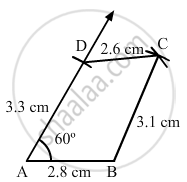Share

# Construct a Quadrilateral Abcd in Which Ab = 2.8 Cm, Bc = 3.1 Cm, Cd = 2.6 Cm, and Da = 3.3 Cm and ∠A = 60°. - Mathematics

Course
ConceptConstructing a Quadrilateral When Two Adjacent Sides and Three Angles Are Known

#### Question

Construct a quadrilateral ABCD in which AB = 2.8 cm, BC = 3.1 cm, CD = 2.6 cm, and DA = 3.3 cm and ∠A = 60°.

#### Solution

Steps of construction:

Step I: Draw AB = 2 . 8 cm .

Step II: Construct ∠BAD = 60° .

Step III : With A as the centre and radius 3 . 3 cm, cut off AD = 3 . 3 cm .

Step IV: With D as the centre and radius 2 . 6 cm, draw an arc .

Step V : With B as the centre and radius 3 . 1 cm, draw an arc to intersect the arc drawn in Step IV at C .

Step VI: Join BC and CD to obtained the required quadrilateral .Is there an error in this question or solution?

#### APPEARS IN

RD Sharma Solution for Mathematics for Class 8 by R D Sharma (2019-2020 Session) (2017 to Current)
Chapter 18: Practical Geometry (Constructions)
Ex. 18.3 | Q: 5 | Page no. 8
Solution Construct a Quadrilateral Abcd in Which Ab = 2.8 Cm, Bc = 3.1 Cm, Cd = 2.6 Cm, and Da = 3.3 Cm and ∠A = 60°. Concept: Constructing a Quadrilateral - When Two Adjacent Sides and Three Angles Are Known.
S